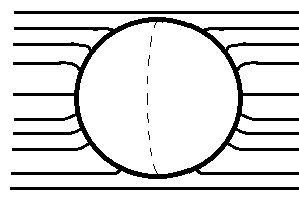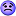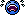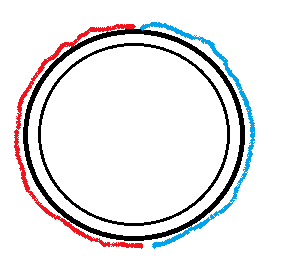# A conducting shell kept in a uniform electric field

• Titan97
In summary, the force that tries to rip apart a conducting shell into two halves about its equator when kept in a uniform electric field of magnitude EEE is repulsive.f

Gold Member

## Homework Statement

Find the force that tries to rip apart a conducting shell into two halves about its equator when kept in a uniform electric field of magnitude ##E##.## Homework Equations

Gauss Law: $$\nabla\cdot\vec{E}=\frac{\rho}{\epsilon_0}$$
I think Laplace equation might also be helpful.

## The Attempt at a Solution

Firstly, why should the two halves repel each other?

Can you just help me to figure out that part? Then I will attempt the question once more.

I think there will be an induced polarisation on the shell, won't it?
If the field lines go from left to right, the electric field should attract positive charges to the right side of the shell and negative ones to the left. In this case, the two sides repell each other. Or am I wrong?

•Titan97
Find the force that tries to rip apart a conducting shell into two halves about its equator when kept in a uniform electric field of magnitude EEE.

If you wish to calculate the force that tries to rip apart the hemispheres- it means that the two halves are being attracyed to each other,
why?
the electric field must have done some displacement of the charges on the conducting shell and if the hemisphers have got charges then it must have some potential developed at the surface..
let us try to model the system by imaginary cutting the spherical conductor by a plane.

•Titan97
@drvrm rip apart means to repel. If the two halves attract each other, they will stick to each other.

I think there will be an induced polarisation on the shell, won't it?
If the field lines go from left to right, the electric field should attract positive charges to the right side of the shell and negative ones to the left. In this case, the two sides repell each other. Or am I wrong?
You are right about the induced charges being positive one side and negative the other, but I believe opposite charges attract. What other reason might there be a force pulling them apart?

•Alettix and Titan97
@drvrm rip apart means to repel. If the two halves attract each other, they will stick to each other.

actually there can be two types of force - the external electric field acting on the hemispherical shells as well as due tp the effective displacement of the charges leading to a dipole field equivalent to the two hemispherical charge distribution and the net force can be repulsive .

•Titan97
@drvrm can you explain in simple language?

@drvrm can you explain in simple language?

the external electric field will contribute to a potential at any point outside the spherical conductor say at P(r. theta) ; however the displacement of charges of the conductor (induced charges) can be looked as equivalent to a dipole placed at the centre of the conductor and its potential contribution at P can be estimated. these potentials can have different nature and
the electric field intensity due to these two may have a net repulsion between the hemispheres

•Titan97ok. I just saw a problem in purcell and morin's asking me to prove that it forms a dipole. I am going to go through it.ok. I just saw a problem in purcell and morin's asking me to prove that it forms a dipole. I am going to go through it.
I'm not so sure the dipole is relevant. The dipole results from the charges on both hemispheres, but the force on one hemisphere does not involve the field its own charges generate. It comes from its own charge in relation to the fields from the other hemisphere and the background field.
However, I do not yet have any ideas on how you would quantify it.

•Titan97
@haruspex what force is responsible for repulsion?

@haruspex what force is responsible for repulsion?
Don't think of it specifically as repulsion. Just think what forces act on each hemisphere.

•Titan97
ok. I just saw a problem in purcell and morin's asking me to prove that it forms a dipole. I am going to go through it.

you can consult/see the problem given in Jackson
i have got a reference -i do not know it is correct view but a dipole has been used in the method of images-
ref;
http://faculty.uml.edu/cbaird/all_homework_solutions/Jackson_2_9_Homework_Solution.pdf

•Titan97
Why are they letting ##Q\to\infty##?

Why are they letting Q→∞Q→∞Q\to\infty?

i think they are trying to portray a field as Q tends to infinity R also tends to infinity giving Q/R.^2.. finite actually this field is responsible for induced charges in conductor later they will relate it to field intensity E(0)

as finally they have to calculate E-field on the surface of the conductor and derive the charge density then can finally calculate force between two hemispheres.

•Titan97
•Titan97
As posts 16 and 17 allude, the problem can be set up as a dipole of charge -q and +q centered at the center of the shell and collinear with the external E field.

The external E field can in turn be modeled by two charges Q and -Q placed distances -d and +d away from the shell's center. The dipole charges are then immediately determined in magnitude, polarity and position inside the shell.

The ensuing picture is that of 4 charges: the +Q charge pulls harder on the -q charge than it pushes on the +q charge. Similarly, the -Q charge pulls more heavily on the +q charge than it repels the -q charge. The net effect is to exert a force repelling the two shell halves from each other.

•Titan97 and drvrm
@haruspex due to the electric field, the charge distribution will be like this right?red represents negative charge and blue represents positive charge.
Hence the left part tries to move left and the right part tries to move right. (repulsion)

The field inside the shell will be canceled out the external field (clarification needed).

I can find the surface charge density by using the solution of Laplace equation in spherical coordinates.

If this method is correct, I will reply the surface charge density appearing on the shell as a function of ##\theta##.

@haruspex due to the electric field, the charge distribution will be like this right?
View attachment 99214
red represents negative charge and blue represents positive charge.
Hence the left part tries to move left and the right part tries to move right. (repulsion)
Yes this is in line with my post
The field inside the shell will be canceled out the external field (clarification needed).

Yes, the two fields cancel each other inside the shell. We know there is zero E field in the metallic shell itself and since there is no surface charge on the inside of the shell the change in E must be zero as we enter the inside of the shell.
I can find the surface charge density by using the solution of Laplace equation in spherical coordinates.
If this method is correct, I will reply the surface charge density appearing on the shell as a function of ##\theta##.
This sounds difficult. And even if you succeed in finding the surface charge density, which BTW will be a function of spherical coordinates θ and φ, not just θ, how will you then determine the forces pulling the two halves apart? I think the image method is a lot easier ...

•Titan97
Once I know the charge on the shell, then I will find force using $$F=\int Edq$$ I will post my attempt soon. Even if method of images is easier, I want to solve this problem in more than one way.

The field inside the shell will be canceled out the external field (clarification needed).

I can find the surface charge density by using the solution of Laplace equation in spherical coordinates.

If this method is correct, I will reply the surface charge density appearing on the shell as a function of θθ\theta.
if you have surface charge density and the electric field at the surface ,one can calculate the force ...you may try ans see.

•Titan97
Hence the left part tries to move left and the right part tries to move right. (repulsion)
Your use of the word repulsion suggests to me that you think the positive charge on one half repels the negative charge on the other. As I reminded Alettix, opposite charges attract. I know @rude man said yes to this, but I don't think he could have realized exactly what you were implying.
Your diagram is missing the external field. What is the affect of that on each half?

•Titan97
Your use of the word repulsion suggests to me that you think the positive charge on one half repels the negative charge on the other. As I reminded Alettix, opposite charges attract. I know @rude man said yes to this, but I don't think he could have realized exactly what you were implying.
Your diagram is missing the external field. What is the affect of that on each half?
In setting up the four charges I described previously, some of the forces are indeed attractive as Alettix described, but they get overshadowed by the greater repulsive forces. It's a matter of what direction the net forces effect.

•Titan97
In setting up the four charges I described previously, some of the forces are indeed attractive as Alettix described, but they get overshadowed by the greater repulsive forces. It's a matter of what direction the net forces effect.
I still object to the use of the word repulsion here. What, exactly, is repelling one hemisphere? The diagram in post #20 only shows the hemispheres, no external field, and no point charges standing in for the external field.

•Titan97
I still object to the use of the word repulsion here. What, exactly, is repelling one hemisphere? The diagram in post #20 only shows the hemispheres, no external field, and no point charges standing in for the external field.
Did you mean post 19? Or 1?
Post 1 shows the external field (the straight lines); the presence of the induced charges is manifest by the orthogonality of the E field lines directly against the shell's surface tangent. Post 19 just shows the induced surface charges which would not be there were there no incident E field.

As to your question - one hemisphere is repelling the other.

•Titan97
@haruspex the electric field is the one that tries to rip apart the shell.

@haruspex the electric field is the one that tries to rip apart the shell.
Yes.
As to your question - one hemisphere is repelling the other.
No.

•Titan97
Yes.

No.
Right.
I meant to say that the effect is for the two halves to want to move apart from each other, but they are not the agents trying to do so.

The answer is that the E field acts on the induced charges so as to try to pull the two halves apart from each other.

•Titan97
Right.
I meant to say that the effect is for the two halves to want to move apart from each other, but they are not the agents trying to do so.

The answer is that the E field acts on the induced charges so as to try to pull the two halves apart from each other.
Ok, but calling that repulsion is confusing.

•Titan97
Ok, but calling that repulsion is confusing.
I agree, interpreting that the two repel each other is not a correct view.
Once I know the charge on the shell, then I will find force using $$F=\int Edq$$ I will post my attempt soon. Even if method of images is easier, I want to solve this problem in more than one way.
OK, if & when you have a numerical answer I am willing to compare results ...

•Titan97
Solution to lapalace equation in spherical coordinates:

$$\Phi=\sum_{i=0}^{\infty}A_ir^i+B_ir^{-i-1}P_i(\cos\theta)$$

I have no idea what that means. But using the boundary conditions, I evaluated the constants. (I did not understand the derivation. It's beyond my high school syllabus).

• At ##r=a##, $$A_ia^i=-B_ia^{-i-1}$$ $$B_I=A_ia^{2i+1}$$
• At ##r\to\infty## $$-E_0r\cos\theta=\sum_{i=0}^{\infty}A_ir^iP_i(\cos\theta)$$ Now, by equating the coefficients, $$A_1=-E_0$$ since ##P_1(\cos\theta)=\cos\theta##
I am not sure if I can equate the coefficients like in point two. ##x=x^2+2x+3## does not mean that ##1=2##.
Also, at point one, I assumed that ##P_i\cos\theta\neq0##.

How should I proceed?

Last edited:
Ok, but calling that repulsion is confusing.
So stipulated!

•Titan97
Solution to lapalace equation in spherical coordinates:
$$\Phi=\sum_{i=0}^{\infty}A_ir^i+B_ir^{-i-1}P_i(\cos\theta)$$
This is the solution for the axisymmetric case where the potential Φ is not a function of the azimuth angle φ. But here you can't ignore φ; Φ is a function of r, θ and φ. (It can for example easily be shown by symmetry argument that the potential is zero everywhere along a line extending from infinity to the center of the shell with the line being perpendicular to the E field).

The non-axisymmetric case is justifiably referred to as "non-examinable" !

•Titan97